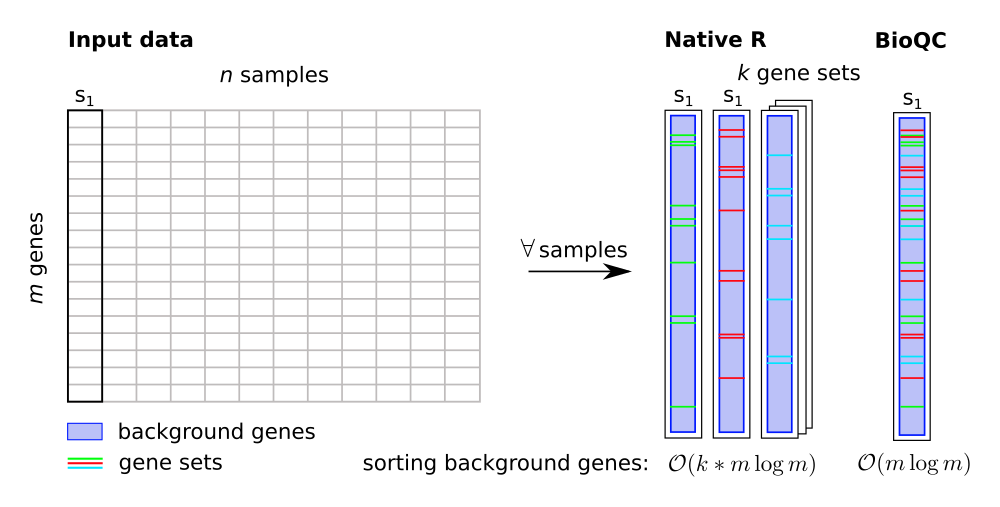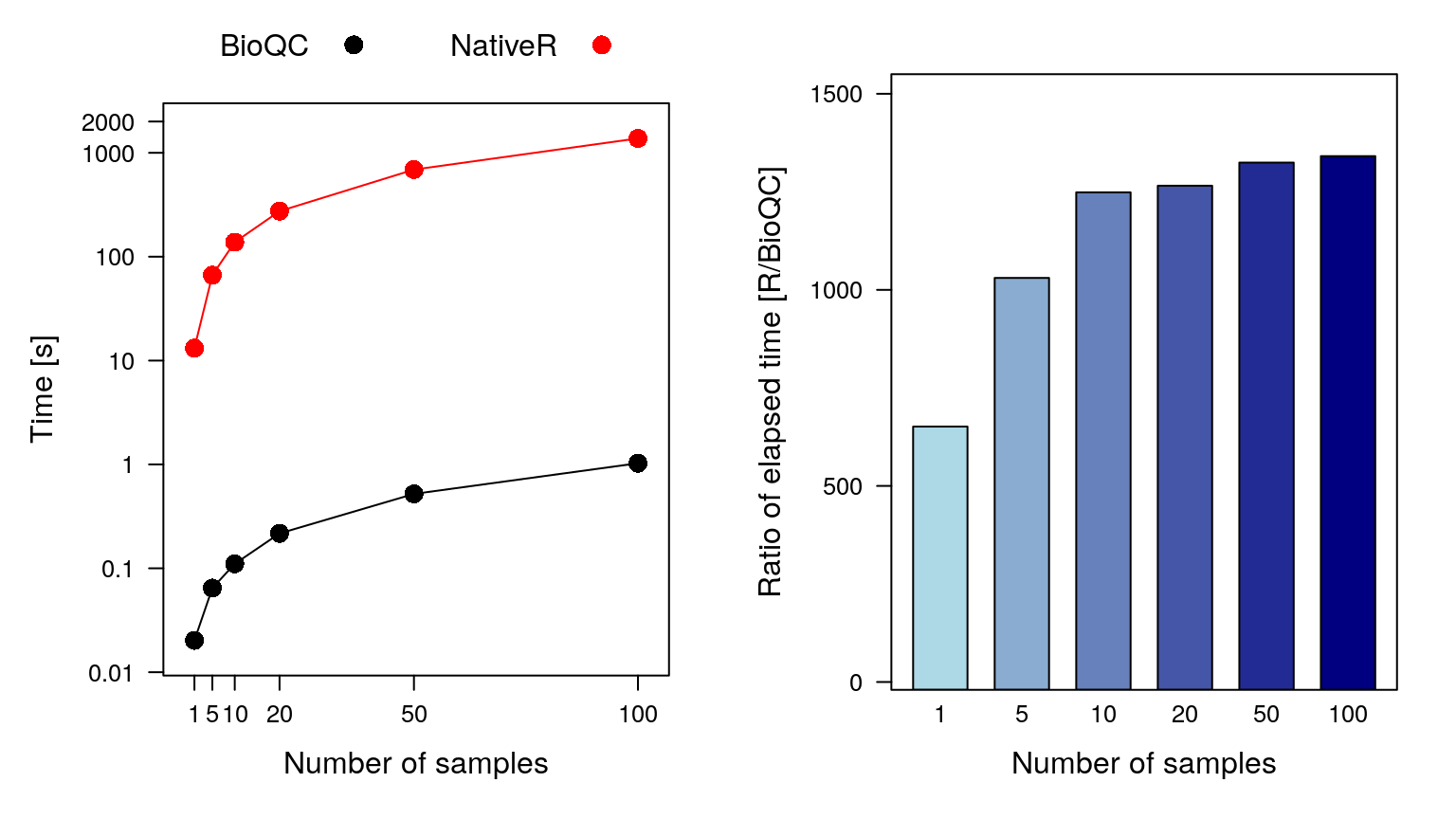In this vignette, we explain the underlaying algorithmic details of our implementation of the Wilcoxon-Mann-Whitney test. The source code used to produce this document can be found in the github repository BioQC.

BioQC is a R/Bioconductor package to detect tissue heterogeneity from high-throughput gene expression profiling data. It implements an efficient Wilcoxon-Mann-Whitney test, and offers tissue-specific gene signatures that are ready to use ‘out of the box’.

## Algorithmic improvements

The Wilcoxon-Mann-Whitney (WMW) test is a non-parametric statistical test to test if median values of two population are equal or not. Unlike the t-test, it does not require the assumption of normal distributions, which makes it more robust against noise.

We improved the computational efficiency of the Wilcoxon-Mann-Whitney test in comparison to the native R implementation based on three modifications:

1. The approximative WMW-statistic (Zar, J. H. (1999). Biostatistical analysis. Pearson Education India. pp. 174-177) is used. The differences to the exact version are negligible for high-throughput gene expression data.
2. The core algorithm is implemented in C instead of R as programming language.
3. BioQC avoids futile expensive sorting operations.

While (1) and (2) are straightforward, we elaborate (3) in the following.

Let $$W_{a,b}$$ be the approximative WMW test of two gene vectors $$a,b$$, where $$a$$ is the gene set of interest, typically containing less than a few hundreds of genes, and $$b$$ is the set of all genes outside the gene set (background genes) typically containing $$>10000$$ genes. In the context of BioQC, the gene sets are referred to as tissue signatures.

Given an $$m \times n$$ input matrix of gene expression data with $$m$$ genes and $$n$$ samples $$s_1, \dots, s_n$$, and $$k$$ gene sets $$d_1, \dots, d_k$$, the WMW-test needs to be applied for each sample $$s_i, i \in 1..n$$ and each gene set $$d_j, j \in 1..k$$. The runtime of the WMW-test is essentially determined by the sorting operation on the two input vectors. Using native R wilcox.test, the vectors $$a$$ and $$b$$ are sorted individually for each gene set. However, in the context of gene set analysis, this is futile, as the (large) background set changes insignificantly in relation to the (small) gene set, when testing different gene sets on the same sample.

Therefore, we approximate the WMW-test by extending $$b$$ to all genes in the sample, keeping the background unchanged when testing multiple gene sets. Like this, $$b$$ has to be sorted only once per sample. The individual gene sets still need to be sorted, which is not a major issue, as they are small in comparison to the set of background genes.BioQC speeds up the Wilcoxon-Mann-Whitney test by avoiding futile sorting operations on the same sample.

## Time benchmark

To demonstrate BioQC’s superior performance, we apply both BioQC and the native R wilcox.test to random expression matrices and measure the runtime.

We setup random expression matrices of 62044 human genes of 1, 5, 10, 50, or 100 samples. Genes are $$i.i.d$$ distributed following $$\mathcal{N}(0,1)$$. The native R and the BioQC implementations of the Wilcoxon-Mann-Whitney test are applied to the matrices respectively.

The numeric results of both implementations, bioqcNumRes (from BioQC) and rNumRes (from R), are equivalent, as shown by the next command.

expect_equal(bioqcNumRes, rNumRes)

The BioQC implementation is more than 500 times faster: while it takes about one second for BioQC to calculate enrichment scores of all 155 signatures in 100 samples, the native R implementation takes about 20 minutes:Figure 2: Time benchmark results of BioQC and R implementation of Wilcoxon-Mann-Whitney test. Left panel: elapsed time in seconds (logarithmic Y-axis). Right panel: ratio of elapsed time by two implementations. All results achieved by a single thread on in a RedHat Linux server.

## Conclusion

We have shown that BioQC achieves identical results as the native implementation in two orders of magnitude less time. This renders BioQC a highly efficient tool for quality control of large-scale high-throughput gene expression data.

## R Session Info

sessionInfo()
## R version 4.1.0 (2021-05-18)
## Platform: x86_64-pc-linux-gnu (64-bit)
## Running under: Ubuntu 20.04.2 LTS
##
## Matrix products: default
##
## locale:
##   LC_CTYPE=en_US.UTF-8       LC_NUMERIC=C
##   LC_TIME=en_US.UTF-8        LC_COLLATE=en_US.UTF-8
##   LC_MONETARY=en_US.UTF-8    LC_MESSAGES=C
##   LC_PAPER=en_US.UTF-8       LC_NAME=C
##  LC_MEASUREMENT=en_US.UTF-8 LC_IDENTIFICATION=C
##
## attached base packages:
##  stats4    stats     graphics  grDevices utils     datasets  methods
##  base
##
## other attached packages:
##   rbenchmark_1.0.0     gplots_3.1.1         gridExtra_2.3
##   latticeExtra_0.6-29  lattice_0.20-44      org.Hs.eg.db_3.13.0
##   AnnotationDbi_1.55.1 IRanges_2.27.2       S4Vectors_0.31.1
##  BioQC_1.21.4         Biobase_2.53.0       BiocGenerics_0.39.2
##  testthat_3.0.4       knitr_1.33
##
## loaded via a namespace (and not attached):
##   Rcpp_1.0.7             locfit_1.5-9.4         png_0.1-7
##   Biostrings_2.61.2      gtools_3.9.2           rprojroot_2.0.2
##   digest_0.6.27          R6_2.5.0               GenomeInfoDb_1.29.3
##  RSQLite_2.2.7          evaluate_0.14          httr_1.4.2
##  highr_0.9              zlibbioc_1.39.0        rlang_0.4.11
##  jquerylib_0.1.4        blob_1.2.2             rmarkdown_2.10
##  pkgdown_1.6.1.9001     textshaping_0.3.5      desc_1.3.0
##  stringr_1.4.0          RCurl_1.98-1.3         bit_4.0.4
##  compiler_4.1.0         xfun_0.25              pkgconfig_2.0.3
##  systemfonts_1.0.2      htmltools_0.5.1.1      KEGGREST_1.33.0
##  GenomeInfoDbData_1.2.6 edgeR_3.35.0           withr_2.4.2
##  crayon_1.4.1           bitops_1.0-7           grid_4.1.0
##  jsonlite_1.7.2         gtable_0.3.0           DBI_1.1.1
##  magrittr_2.0.1         KernSmooth_2.23-20     stringi_1.7.3
##  cachem_1.0.5           XVector_0.33.0         fs_1.5.0
##  limma_3.49.4           bslib_0.2.5.1          ragg_1.1.3
##  vctrs_0.3.8            RColorBrewer_1.1-2     tools_4.1.0
##  memoise_2.0.0          sass_0.4.0